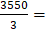# The following question consists of two statements I and II are given below. You have to decide whether the data provided in the statements are sufficient to answer the question. What is the average monthly income per family members? I. Each male earns Rs. 1250 a month and each female earns Rs. 1050 a month. II. Ratio of males to females in the family is 2 : 1 (a) The data in statement I alone was sufficient to the answer the question while II alone not sufficient to the answer the question. (b) Data in statement II alone are sufficient to answer the question while data in statement I alone are not sufficient to answer the questions. (c) The data in statement I alone or in statement II alone are sufficient to answer the questions.            (d) The data in both statements I and II are sufficient to answer the question.

## Question ID - 51452 :- The following question consists of two statements I and II are given below. You have to decide whether the data provided in the statements are sufficient to answer the question. What is the average monthly income per family members? I. Each male earns Rs. 1250 a month and each female earns Rs. 1050 a month. II. Ratio of males to females in the family is 2 : 1 (a) The data in statement I alone was sufficient to the answer the question while II alone not sufficient to the answer the question. (b) Data in statement II alone are sufficient to answer the question while data in statement I alone are not sufficient to answer the questions. (c) The data in statement I alone or in statement II alone are sufficient to answer the questions.            (d) The data in both statements I and II are sufficient to answer the question.

3537

From both I and II, we have:

Let the number of males and females in the family beandrespectively.

Then, average monthly income per member = Rs.= Rs.Rs. 1183.33

So, both I and II together give the answer.

Next Question :

The figure shows a square loop L of side 5 cm which is connected to a network of resistances. The whole setup is moving towards right with a constant speed of 1 cm s–1. At some instant, a part of L is in a uniform magnetic field of 1 T, perpendicular to the plane of the loop. If the resistance of L is 1.7, the current in the loop at that instant will be close to:a) 170b) 60c) 150d) 115